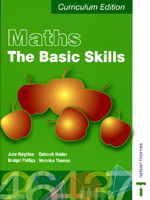Maths
The Basic Skills

By June Haighton, et al.
August 2004
Nelson Thornes
ISBN: 0748777008463 pages, 5 ¼ x 7 ¾"
\$37.50 Paper Original

This new set of resources, comprising three Worksheet Packs and a textbook, have been designed specifically for the new Adult Numeracy Curriculum, covering Entry Levels 1, 2 and 3 and Levels 1 and 2. All topics within the resources are clearly labelled with a curriculum reference to assist with planning.

• The textbook targets the higher levels of the Adult Numeracy Curriculum, Entry Level 3, Level 1 and Level 2. Covering all of the three subject areas of the curriculum in one book, with revision of Entry Level 1 and 2 topics where appropriate.
• Exercises progress from simple numerical questions gradually increasing in difficulty to incorporate numbers into language.
• Suggestions for alternative methods of learning are provided for students who are struggling to comprehend a particular topic.

Contents:
Section 1. Numbers
Chapter 1. Number Value
Chapter 3. Multiplication and Division
Chapter 4. Rounding and Estimation
Chapter 5. Ration and Proportion
Chapter 6. Using Formulae
Chapter 7. Mixed Operations and Calculator Practice
Chapter 8. Fractions
Chapter 9. Decimals
Chapter 10. Percentages

Section 2. Measures, Shape and Space
Chapter 1. Money
Chapter 2. Time
Chapter 3. Length
Chapter 4. Weight
Chapter 5. Capacity
Chapter 6. Temperature
Chapter 7. Perimeter, Area and Volume
Chapter 8. Shape

Section 3. Handling Data
Chapter 1. Extracting and Interpreting Information
Chapter 2. Collecting and Illustrating Data
Chapter 3. Averages and Range
Chapter 4. Probability